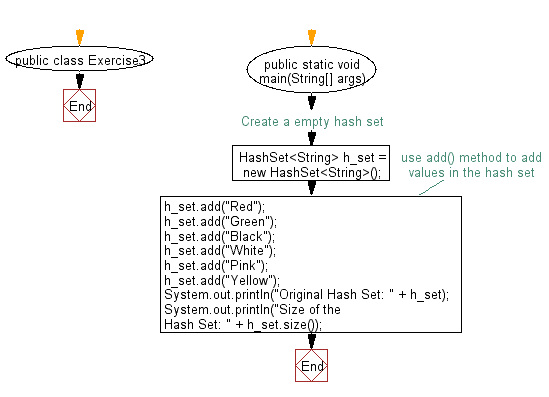﻿ Java: Get the number of elements in a hash set# Java Collection, HashSet Exercises: Get the number of elements in a hash set

## Java Collection, HashSet Exercises: Exercise-3 with Solution

Write a Java program to get the number of elements in a hash set.

Sample Solution:

Java Code:

``````import java.util.*;
import java.util.Iterator;
public class Exercise3 {
public static void main(String[] args) {
// Create a empty hash set
HashSet<String> h_set = new HashSet<String>();
System.out.println("Original Hash Set: " + h_set);
System.out.println("Size of the Hash Set: " + h_set.size());
}
}
``````

Sample Output:

```Original Hash Set: [Red, White, Pink, Yellow, Black, Green]
Size of the Hash Set: 6 ```

Pictorial Presentation:Flowchart:Java Code Editor:

What is the difficulty level of this exercise?

Test your Programming skills with w3resource's quiz.

﻿

## Java: Tips of the Day

getEnumMap

Converts to enum to Map where key is the name and value is Enum itself.

```public static <E extends Enum<E>> Map<String, E> getEnumMap(final Class<E> enumClass) {
return Arrays.stream(enumClass.getEnumConstants())
.collect(Collectors.toMap(Enum::name, Function.identity()));
}
```

Ref: https://bit.ly/3xXcFZt